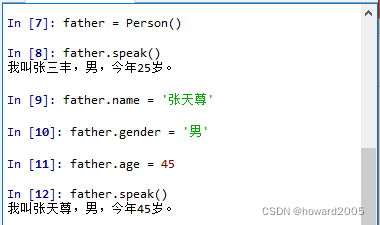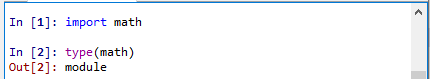# Python編程基礎之類和對象

• 瞭解面向對象編程思想
• 掌握類和對象的定義和使用
• 瞭解Python中的對象

## 一、面向對象

### （一）程序員“面向對象”### （二）兩種編程思想實現五子棋## 二、類和對象

### （一）類和對象的關系

#### 2、示意圖### （二）類的定義

#### 1、類的構成

• 類的名稱：類名，首字母必須大寫，比如Person
• 類的屬性：一組數據，比如姓名、性別、年齡……（描述類的特征 – 靜態）
• 類的方法：允許進行操作的方法，比如說話、步行、跑步……（描述類的行為 – 動態）

#### 2、類定義語法格式

```class 類名:
類的屬性
類的方法
```

```class 類名(父類1, 父類2, …, 父類n):
類的屬性
類的方法
```

#### 3、案例演示### （三）創建對象

#### 1、語法格式

• 利用無參構造方法創建對象：對象名 = 類名()
• 利用有參構造方法創建對象：對象名 = 類名(參數列表)

#### 3、案例演示• 由此可見，在Person類裡定義三個屬性name、gender、age，所有基於該類的對象都具有同樣的初始值，然後可以根據需要修改對象的屬性值。
• 基於Person類再創建一個對象father，調用speak()方法，修改對象屬性值，再調用speak()方法## 三、Python中的對象

### （一）概述

• 在前面，我們已使用過多種對象：整數對象、小數對象、字符串對象、函數對象、模塊對象等。
• 用dir()函數查看一個對象有哪些屬性與方法

### （二）常用對象

#### 1、整數對象

（1）查看整數對象的屬性和方法

```['__abs__',
'__and__',
'__bool__',
'__ceil__',
'__class__',
'__delattr__',
'__dir__',
'__divmod__',
'__doc__',
'__eq__',
'__float__',
'__floor__',
'__floordiv__',
'__format__',
'__ge__',
'__getattribute__',
'__getnewargs__',
'__gt__',
'__hash__',
'__index__',
'__init__',
'__init_subclass__',
'__int__',
'__invert__',
'__le__',
'__lshift__',
'__lt__',
'__mod__',
'__mul__',
'__ne__',
'__neg__',
'__new__',
'__or__',
'__pos__',
'__pow__',
'__rand__',
'__rdivmod__',
'__reduce__',
'__reduce_ex__',
'__repr__',
'__rfloordiv__',
'__rlshift__',
'__rmod__',
'__rmul__',
'__ror__',
'__round__',
'__rpow__',
'__rrshift__',
'__rshift__',
'__rsub__',
'__rtruediv__',
'__rxor__',
'__setattr__',
'__sizeof__',
'__str__',
'__sub__',
'__subclasshook__',
'__truediv__',
'__trunc__',
'__xor__',
'as_integer_ratio',
'bit_length',
'conjugate',
'denominator',
'from_bytes',
'imag',
'numerator',
'real',
'to_bytes']
```

（2）案例演示327的二進制位數是9，調用bin()函數將其轉換成二進制：101000111（9位）#### 2、小數對象

（1）查看小數對象的屬性和方法

```['__abs__',
'__bool__',
'__class__',
'__delattr__',
'__dir__',
'__divmod__',
'__doc__',
'__eq__',
'__float__',
'__floordiv__',
'__format__',
'__ge__',
'__getattribute__',
'__getformat__',
'__getnewargs__',
'__gt__',
'__hash__',
'__init__',
'__init_subclass__',
'__int__',
'__le__',
'__lt__',
'__mod__',
'__mul__',
'__ne__',
'__neg__',
'__new__',
'__pos__',
'__pow__',
'__rdivmod__',
'__reduce__',
'__reduce_ex__',
'__repr__',
'__rfloordiv__',
'__rmod__',
'__rmul__',
'__round__',
'__rpow__',
'__rsub__',
'__rtruediv__',
'__set_format__',
'__setattr__',
'__sizeof__',
'__str__',
'__sub__',
'__subclasshook__',
'__truediv__',
'__trunc__',
'as_integer_ratio',
'conjugate',
'fromhex',
'hex',
'imag',
'is_integer',
'real']
```

（2）案例演示#### 3、字符串對象

（1）查看字符串對象的屬性和方法

```['__add__',
'__class__',
'__contains__',
'__delattr__',
'__dir__',
'__doc__',
'__eq__',
'__format__',
'__ge__',
'__getattribute__',
'__getitem__',
'__getnewargs__',
'__gt__',
'__hash__',
'__init__',
'__init_subclass__',
'__iter__',
'__le__',
'__len__',
'__lt__',
'__mod__',
'__mul__',
'__ne__',
'__new__',
'__reduce__',
'__reduce_ex__',
'__repr__',
'__rmod__',
'__rmul__',
'__setattr__',
'__sizeof__',
'__str__',
'__subclasshook__',
'capitalize',
'casefold',
'center',
'count',
'encode',
'endswith',
'expandtabs',
'find',
'format',
'format_map',
'index',
'isalnum',
'isalpha',
'isascii',
'isdecimal',
'isdigit',
'isidentifier',
'islower',
'isnumeric',
'isprintable',
'isspace',
'istitle',
'isupper',
'join',
'ljust',
'lower',
'lstrip',
'maketrans',
'partition',
'replace',
'rfind',
'rindex',
'rjust',
'rpartition',
'rsplit',
'rstrip',
'split',
'splitlines',
'startswith',
'strip',
'swapcase',
'title',
'translate',
'upper',
'zfill']
```

（2）案例演示#### 4、函數對象#### 5、模塊對象### （三）兩種對象

#### 1、類對象

• Python執行class語句時創建一個類對象和一個變量（名稱就是類名稱），變量引用類對象。與def類似，class也是可執行語句。導入類模塊時，class語句被執行，創建類對象。
• 類中的賦值語句創建的變量是類的數據屬性。與模塊類似，類中的頂層賦值語句創建的變量才屬於類對象。類的數據數據用“對象名.屬性名”格式來訪問。
• 類中的def語句定義的函數是類的方法屬性，用“對象名.方法名()”格式來訪問。

#### 2、實例對象

• 實例對象通過調用類對象來創建（就像調用函數一樣來調用類對象）。
• 每個實例對象繼承類對象的屬性，並獲得自己的命名空間。
• 實例對象的“私有”屬性。類的方法函數的第一個參數默認為self，表示引用方法的對象實例。在方法中對self的屬性賦值才會創建屬於實例對象的屬性。